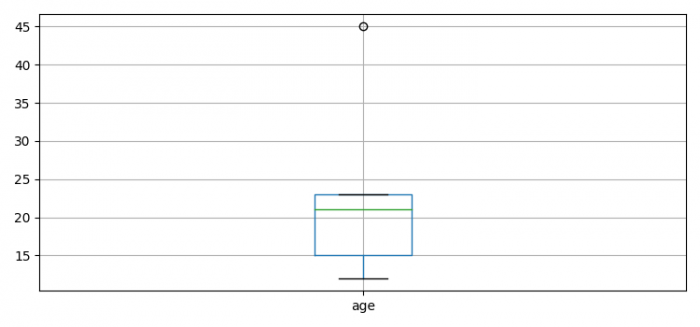# How to get boxplot data for Matplotlib boxplots?

To get boxplot data for Matplotlib boxplot we can take the following steps −

• Set the figure size and adjust the padding between and around the subplots.
• Make data frame using pandas.
• Make a box plot from DataFrame columns.
• Get boxplot's outliers, boxes, medians and whiskers data.
• Prit all the above information.
• To display the figure, use show() method.

## Example

import seaborn as sns
import pandas as pd
from matplotlib import pyplot as plt

plt.rcParams["figure.figsize"] = [7.50, 3.50]
plt.rcParams["figure.autolayout"] = True

df = pd.DataFrame(dict(age=[23, 45, 21, 15, 12]))
_, bp = pd.DataFrame.boxplot(df, return_type='both')
outliers = [flier.get_ydata() for flier in bp["fliers"]]
boxes = [box.get_ydata() for box in bp["boxes"]]
medians = [median.get_ydata() for median in bp["medians"]]
whiskers = [whiskers.get_ydata() for whiskers in bp["whiskers"]]

print("Outliers: ", outliers)
print("Boxes: ", boxes)
print("Medians: ", medians)
print("Whiskers: ", whiskers)

plt.show()

## Output

Outliers: [array()]
Boxes: [array([15., 15., 23., 23., 15.])]
Medians: [array([21., 21.])]
Whiskers: [array([15., 12.]), array([23., 23.])]